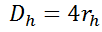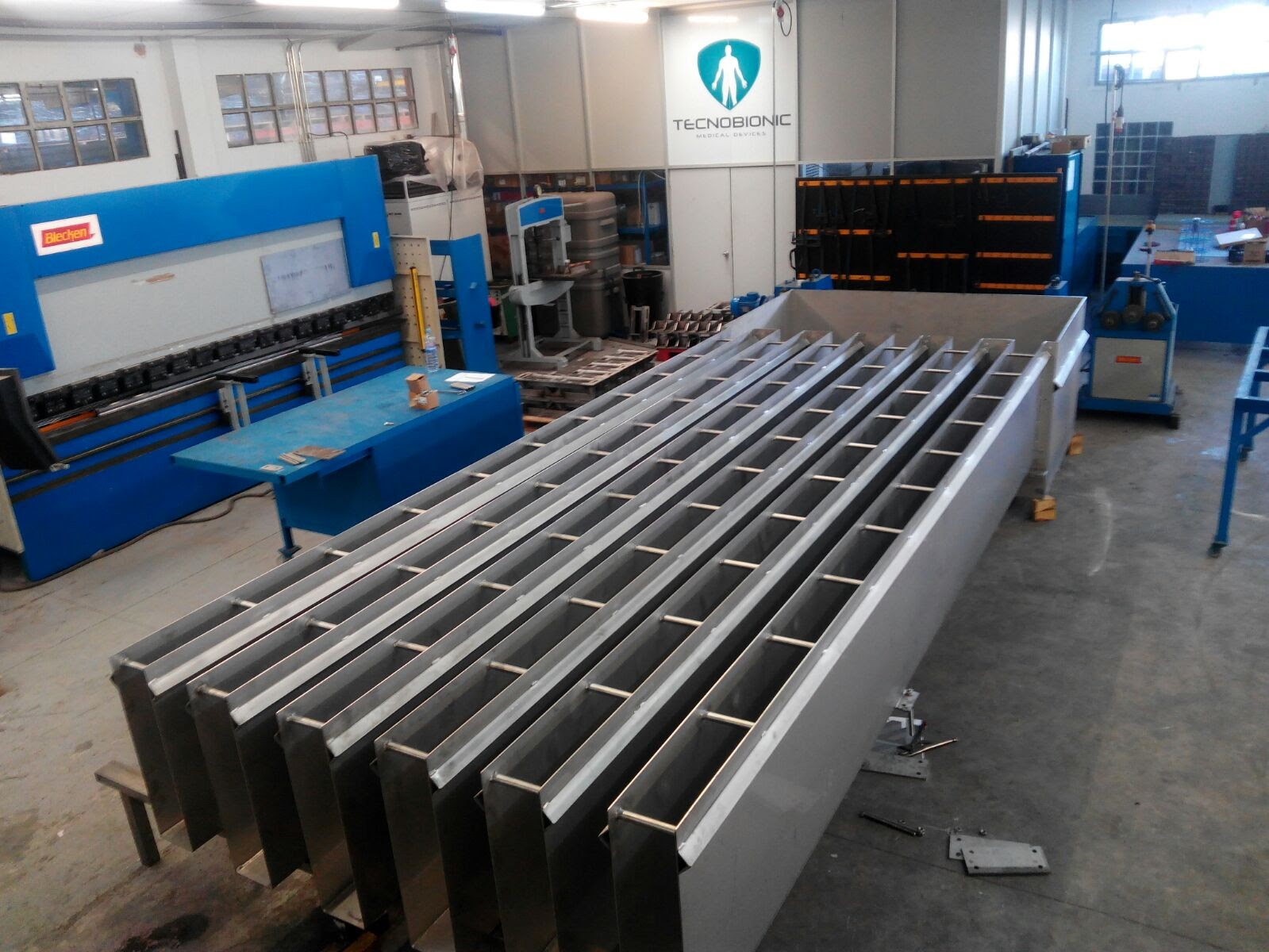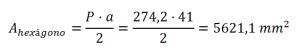Blog Article

What are the hydraulic diameter and radio?

posted in Technical Articles byThe hydraulic diameter is a characteristic parameter of a tube section or generic cannel, and allows studying the flow behavior just as if it was circular.

Where,

–   A is the cross sectional area of the channel

–   P is the humid perimeter

It´s important to note that the hydraulic radius (Rh) is not half the hydraulic diameter. Both parameters relate them self by the following:Example of hydraulic diameter calculation for TecnoTec lamella H80:The hydraulic diameter of a lamella TecnoTec H80 is calculated as follows:Where,and,(a is the apotema)

Replacing values in the hydraulic diameter formula, it is easy to calculate that:06 May, 15